# How to Add Trailing Spaces to Text in Excel?

Sometimes we may have to copy the data from an Excel sheet to a Notepad file. If we directly copy the data in Excel into Notepad, the whole thing will look very clumsy. So, before copying the data, we have to add trailing spaces for every cell in excel, then we can copy the data to notepad. Trailing spaces are nothing but the empty space after the line without any character after the space, so let us see a simple process to add trailing spaces to the Excel cells.

## Add Trailing Spaces to Text in Excel

Here, we will first arrange the data using the LEFT and REPT formulas, then copy the data. Let's take a look at a simple procedure for adding trailing spaces to each cell in Excel.

Step 1

Consider the following Excel sheet with data similar to the image below.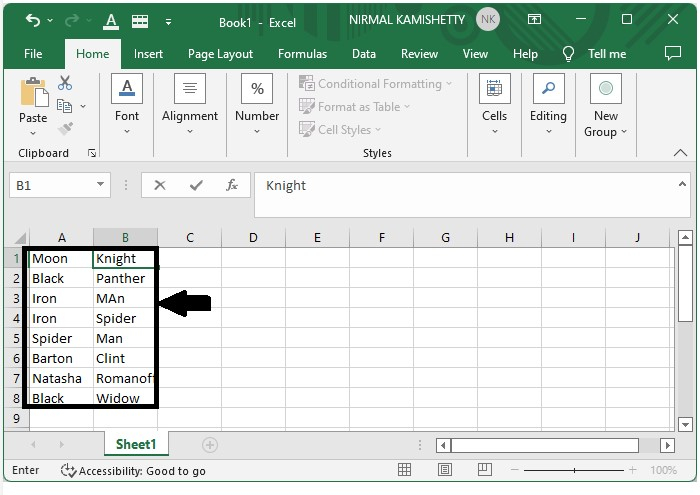Now try to copy the data into a notepad.

To copy the data, select it all in Excel and press CTRL + C. Then, open a notepad and use the command CTRL + V to paste the copied data from Excel on to the notepad, and it will appear as shown in the following image −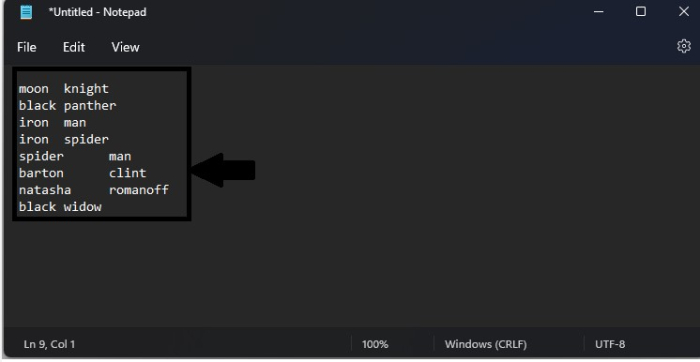As we can see, the data that we pasted directly from Excel is looking messy and is very annoying to understand, so we can use trailing spaces in Excel to make it look tidy.

Step 2

To add trailing spaces to the spreadsheet, click on an empty cell and enter the formula =LEFT(A1REPT("",10),10) in the formula box and click Enter to create our first result cell.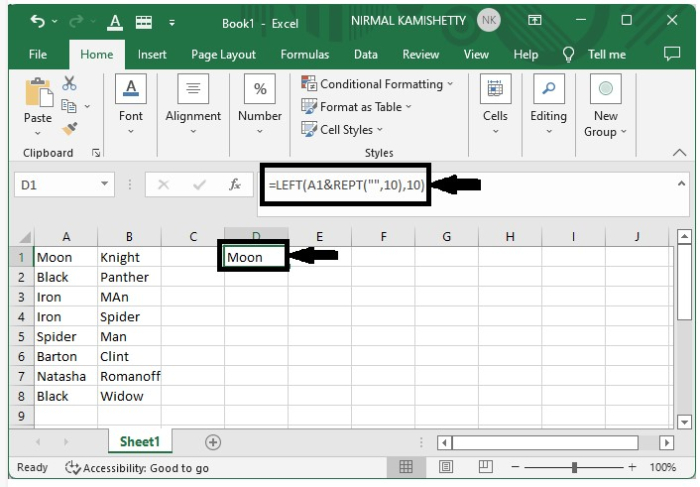Now drag from the right corner of the first result using the auto-fill handle to get all the results.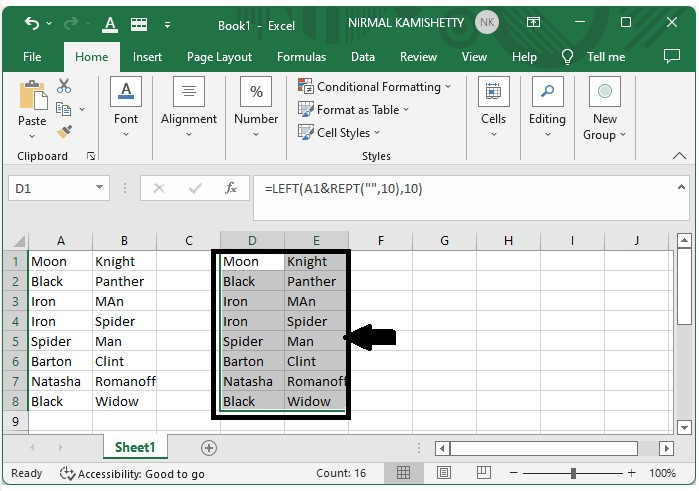Even though we can’t see any difference between the two sets of data, we can see a huge difference when we copy the data into a notepad.

So, let's paste the generated data into notepad and compare the results.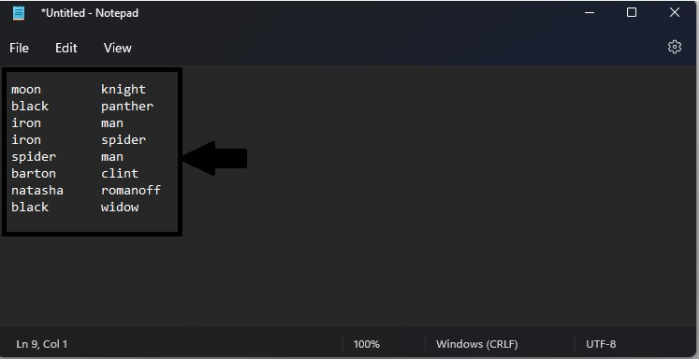## Conclusion

In this tutorial, we used a simple example to demonstrate how you can add trailing spaces to text in Excel to highlight a particular set of data.